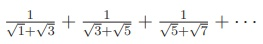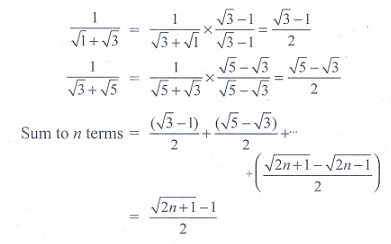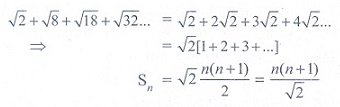Home | | Maths 11th std | Exercise 5.5: Choose the correct answer

# Exercise 5.5: Choose the correct answer

Multiple choice questions with answers / choose the correct answer with answers - Maths Book back 1 mark questions and answers with solution for Exercise Problems

CHAPTER : Binomial Theorem, Sequences And Series

1. The value of 2 + 4 + 6 +…….+ 2n is

(1) n(n-1)/2

(2) n(n+1)/2

(3) 2n(2n+1)/2

(4) n(n + 1)

Solution2. The coefficient of x6 in (2 + 2x)10 is

(1) 10C6

(2) 26

(3) 10C6 26

(4) 10C6 210.

Solution3. The coefficient of x8y12 in the expansion of (2x + 3y)20 is

(1) 0

(2) 28312

(3) 28312 + 21238

(4) 20C8 28312.

Solution4. If nC10 > nCr for all possible r, then a value of n is

(1) 10

(2) 21

(3) 19

(4) 20.

Solution5. If a is the arithmetic mean and g is the geometric mean of two numbers, then

(1) a ≤ g

(2) a ≥ g

(3) a = g

(4) a > g.

Solution6. If (1 + x2)2 (1 + x)n = a0 + a1x + a2x2 +……..+ xn+4 and if a0, a1, a2 are in AP, then n is

(1) 1

(2) 2

(3) 3

(4) 4.

Solution7. If a, 8, b are in AP, a, 4, b are in GP, and if a, x, b are in HP then x is

(1) 2

(2) 1

(3) 4

(4) 16.

Solution8. The sequenceform an

(1) AP

(2) GP

(3) HP

(4) AGP.

Solution9. The HM of two positive numbers whose AM and GM are 16, 8 respectively is

(1) 10

(2) 6

(3) 5

(4) 4.

Solution10. If Sn denotes the sum of n terms of an AP whose common difference is d, the value of Sn - 2Sn-1 + Sn-2 is

(1) 0

(2) 2d

(3) 4d

(4) d2.

11. The remainder when 3815 is divided by 13 is

(1) 12

(2) 1

(3) 11

(4) 5.

Solution12. The nth term of the sequence 1, 2, 4, 7, 11,…….is

(1) n3 + 3n2 + 2n

(2) n3 - 3n2 + 3n

(3) n(n+1)(n+2) / 3

(4) n2-n+2 / 2 .

13. The sum up to n terms of the seriesisAns: 4

Solution14. The nth term of the sequence 1/2 , 3/4 , 7/8 , 15/16 ,……….is

(1) 2n - n - 1

(2) 1 - 2-n

(3) 2-n + n - 1

(4) 2n-1.

Solution15. The sum up to n terms of the series √2 + √8 + √18 + √32 +……….is

(1) n(n+1) / 2

(2) 2n(n + 1)

(3) n(n+1) / 2

(4) 1.

Solution16. The value of the series 1/2 + 7/4 + 13/8 + 19/16 +…………. is

(1) 14

(2) 7

(3) 4

(4) 6.

Solution17. The sum of an infinite GP is 18. If the first term is 6, the common ratio is

(1) 1/3

(2) 2/3

(3) 1/6

(4) 3/4 .

Solution18. The coefficient of x5 in the series e-2x is

(1) 2/3

(2) 3/2

(3) -4/15

(4) 4/15 .

Solution19. The value of 1/2! + 1/4! + 1/6! +……… is

(1) e2+1 / 2e

(2) (e+1)2 / 2e

(3) (e-1)2 / 2e

(4) e2+1 / 2e .

Solution20. The value ofis

(1) log (5/3)

(2) 3/2 log(5/3)

(3) 5/3 log(5/3)

(4) 2/3 log(2/3)

SolutionTags : Binomial Theorem, Sequences and Series | Mathematics , 11th Mathematics : UNIT 5 : Binomial Theorem, Sequences and Series
Study Material, Lecturing Notes, Assignment, Reference, Wiki description explanation, brief detail
11th Mathematics : UNIT 5 : Binomial Theorem, Sequences and Series : Exercise 5.5: Choose the correct answer | Binomial Theorem, Sequences and Series | Mathematics